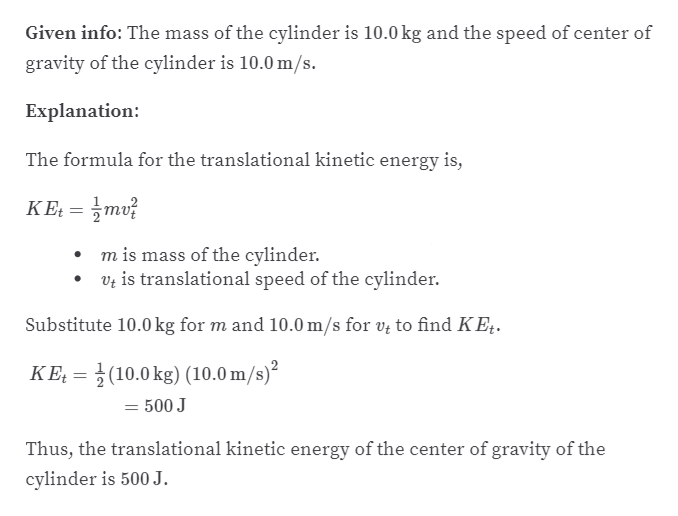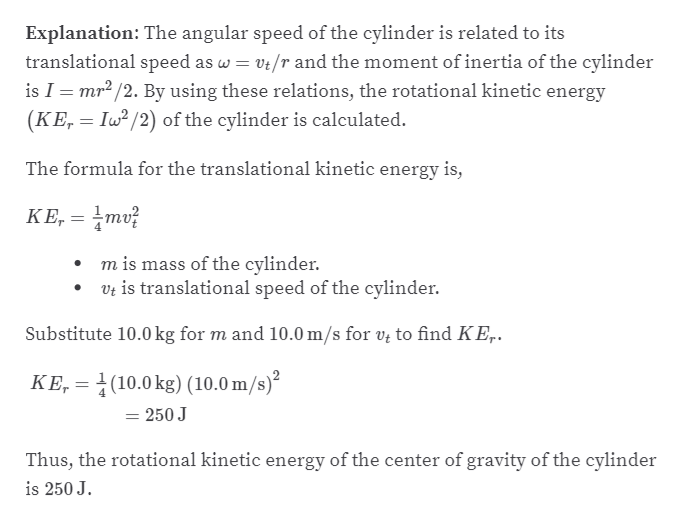# A 10.0- kg cylinder rolls without slipping on a rough surface.At an instant when its center of gravity has a speed of 10.0 m/s,determine (a) the translational kinetic energy of its center ofgravity, (b) the rotational kinetic energy about its center ofgravity, and (c) its total kinetic energy.

Question
1 views

A 10.0- kg cylinder rolls without slipping on a rough surface.
At an instant when its center of gravity has a speed of 10.0 m/s,
determine (a) the translational kinetic energy of its center of
gravity, (b) the rotational kinetic energy about its center of
gravity, and (c) its total kinetic energy.

check_circle

Step 1

(a)help_outlineImage TranscriptioncloseGiven info: The mass of the cylinder is 10.0 kg and the speed of center of gravity of the cylinder is 10.0 m/s. Explanation: The formula for the translational kinetic energy is, KE: = mv? m is mass of the cylinder. Vz is translational speed of the cylinder. Substitute 10.0 kg for m and 10.0 m/s for vų to find KEț. KE, = }(10.0 kg) (10.0 m/s)² = 500 J Thus, the translational kinetic energy of the center of gravity of the cylinder is 500 J. fullscreen
Step 2

(b)

...help_outlineImage TranscriptioncloseExplanation: The angular speed of the cylinder is related to its translational speed as w = vt/r and the moment of inertia of the cylinder is I = mr2 /2. By using these relations, the rotational kinetic energy (KE, = Iw? /2) of the cylinder is calculated. The formula for the translational kinetic energy is, KE, = mv? m is mass of the cylinder. Vt is translational speed of the cylinder. Substitute 10.0 kg for m and 10.0 m/s for v; to find KE,. KE, = (10.0 kg) (10.0 m/s)² = 250 J %3D Thus, the rotational kinetic energy of the center of gravity of the cylinder is 250 J. fullscreen

### Want to see the full answer?

See Solution

#### Want to see this answer and more?

Solutions are written by subject experts who are available 24/7. Questions are typically answered within 1 hour.*

See Solution
*Response times may vary by subject and question.
Tagged in

### Physics